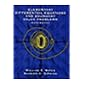Normal view

# Differential equations & linear algebra / C. Henry Edwards, David E. Penney

Material type:TextPublisher: Upper Saddle River, NJ : Pearson Prentice Hall, Copyright date: ℗♭2005Edition: Second editionDescription: xv, 751, 8 pages : color illustrations ; 27 cmContent type: text Media type: unmediated Carrier type: volumeISBN: 0131481460; 9780131481466; 0131984810; 9780131984813Other title: Differential equations and linear algebraSubject(s): Differential equations | Algebras, LinearDDC classification: 515/.35 LOC classification: QA372 | .E34 2005
Contents:
First-Order Differential Equations -- Mathematical Models and Numerical Methods -- Linear Systems and Matrices -- Vector Spaces -- Higher-Order Linear Differential Equations -- Eigenvalues and Eigenvectors -- Linear Systems of Differential Equations -- Matrix Exponential Methods -- Nonlinear Systems and Phenomena -- Laplace Transform Methods -- Power Series Methods
Tags from this library: No tags from this library for this title.
Star ratingsAverage rating: 0.0 (0 votes)
Holdings
Item type Current library Call number Status Date due BarcodeBooks
Second Floor - Engineering & Architecture
515.35 E.C.D 2006 (Browse shelf (Opens below)) Available 23428
##### Browsing Centeral Library shelves, Shelving location: Second Floor - Engineering & Architecture Close shelf browser (Hides shelf browser)515.35 B.W.E 2014 Elementary differential equations and boundary value problems \ 515.35 B.W.E 2014 Elementary differential equations with boundary value problems / 515.35 E.C.D 2004 Differential equations : 515.35 E.C.D 2006 Differential equations & linear algebra / 515.35 J.M.N 2014 Numerical solution of differential equations / 515.35 N.R.F 2014 Fundamentals of differential equations / 515.35 N.R.F 2014 Fundamentals of differential equations /

Revised edition of: Differential equations and linear algebra. c2001

Includes bibliographical references (pages 671-672) and index

First-Order Differential Equations -- Mathematical Models and Numerical Methods -- Linear Systems and Matrices -- Vector Spaces -- Higher-Order Linear Differential Equations -- Eigenvalues and Eigenvectors -- Linear Systems of Differential Equations -- Matrix Exponential Methods -- Nonlinear Systems and Phenomena -- Laplace Transform Methods -- Power Series Methods

There are no comments on this title.